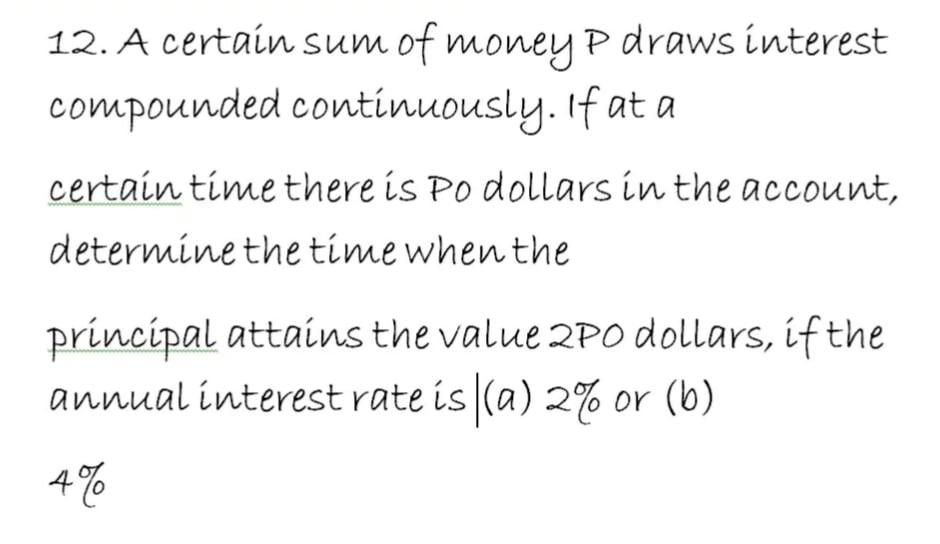# Question Solved1 Answer12. A certain sum of money p draws interest compounded continuously. If at a certain time there is po dollars in the account, determine the time when the principal attains the value 2Po dollars, if the annual interest rate is (a) 2% or (b) 4%Transcribed Image Text: 12. A certain sum of money p draws interest compounded continuously. If at a certain time there is po dollars in the account, determine the time when the principal attains the value 2Po dollars, if the annual interest rate is (a) 2% or (b) 4%
More
Transcribed Image Text: 12. A certain sum of money p draws interest compounded continuously. If at a certain time there is po dollars in the account, determine the time when the principal attains the value 2Po dollars, if the annual interest rate is (a) 2% or (b) 4%